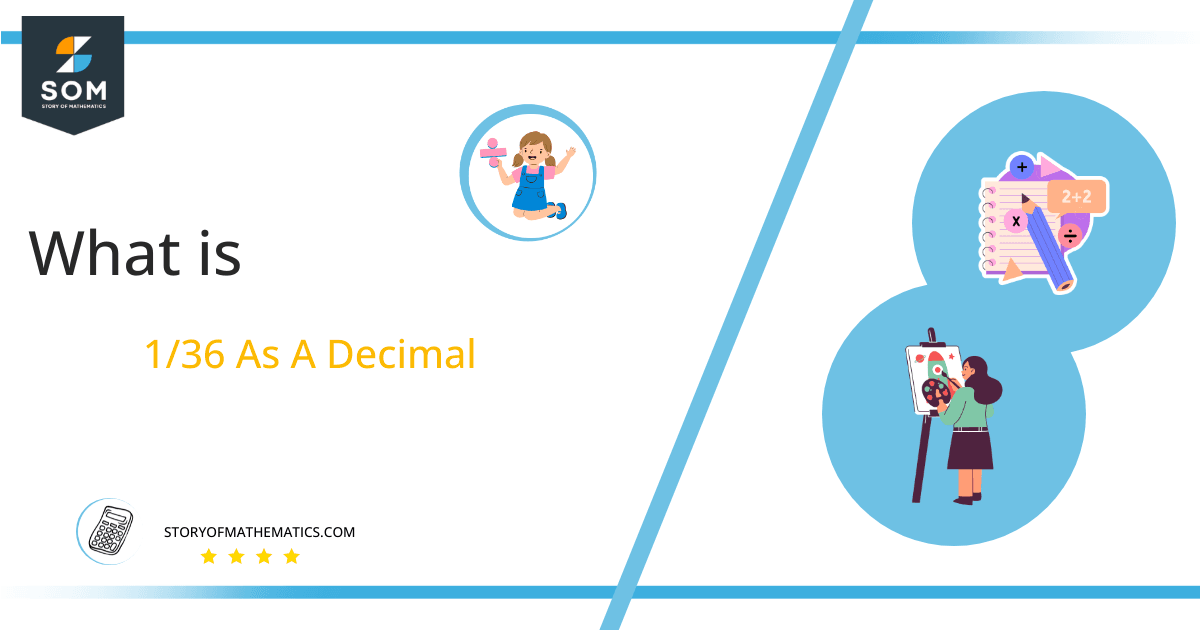# What Is 1/36 as a Decimal + Solution With Free Steps

The fraction 1/36 as a decimal is equal to 0.0277.

The division is one of the four primary operations of mathematics. There are two types of divisions. One solves completely and results in an Integer value, whilst the other doesn’t solve to completion, producing a Decimal value.

Here, we are interested more in the types of division that results in a Decimal value, as this can be expressed as a Fraction. We see fractions as a way of showing two numbers having the operation of Division between them that result in a value that lies between two Integers.Now, we introduce the method used to solve said fraction to decimal conversion, called Long Division which we will discuss in detail moving forward. So, let’s go through the Solution of fraction 1/36.

## Solution

First, we convert the fraction components i.e., the numerator and the denominator, and transform them into the division constituents i.e., the Dividend and the Divisor respectively.

This can be seen done as follows:

Dividend = 1

Divisor = 36

Now, we introduce the most important quantity in our process of division, this is the Quotient. The value represents the Solution to our division, and can be expressed as having the following relationship with the Division constituents:

Quotient = Dividend $\div$ Divisor = 1 $\div$ 36

This is when we go through the Long Division solution to our problem. Following is the figure showing division:## 1/36 Long Division Method

We start solving a problem using the Long Division Method by first taking apart the division’s components and comparing them. As we have 1, and 36 we can see how 1 is Smaller than 36, and to solve this division we require that 1 be Bigger than 36.

This is done by multiplying the dividend by 10 or 100 depending on the decimal position and checking whether it is bigger than the divisor or not. If it is then we calculate the Multiple of the divisor which is closest to the dividend and subtract it from the Dividend. This produces the Remainder which we then use as the dividend later.

Now, we begin solving for our dividend 1, which after getting multiplied by 10 becomes 10 that is still smaller than 36 therefore we will multiply it again with 10 to make it 100.

We take this 100 and divide it by 36, this can be seen done as follows:

100 $\div$ 36 $\approx$ 2

Where:

36 x 2 = 72

This will lead to the generation of a Remainder equal to 100 – 72 = 28, now this means we have to repeat the process by Converting the 28 into 280 and solving for that:

280 $\div$ 36 $\approx$ 7

Where:

36 x 7 = 252

This, therefore, produces another remainder which is equal to 280 – 252 = 28. Now we must solve this problem to Third Decimal Place for accuracy, so we repeat the process with dividend 280.

280 $\div$ 7 $\approx$ 252

Where:

37 x 7 = 252

Finally, we have a Quotient generated after combining the three pieces of it as 0.0277 = z, with a Remainder equal to 28.Images/mathematical drawings are created with GeoGebra.